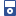Accessibility options:

# Simple functions, function notation, & graphs resources

Show me all resources applicable to

### iPOD Video (6)Introduction to Functions - Part 1
IPOD VIDEO: A function is a rule which operates on one number to give another number. However, not every rule describes a valid function. This unit explains how to see whether a given rule describes a valid function, and introduces some of the mathematical terms associated with functions. This resource is released under a Creative Commons license Attribution-Non-Commercial-No Derivative Works and the copyright is held by Skillbank Solutions Ltd.Introduction to Functions - Part 2
IPOD VIDEO: A function is a rule which operates on one number to give another number. However, not every rule describes a valid function. This unit explains how to see whether a given rule describes a valid function, and introduces some of the mathematical terms associated with functions. This resource is released under a Creative Commons license Attribution-Non-Commercial-No Derivative Works and the copyright is held by Skillbank Solutions Ltd.Introduction to Functions - Part 3
IPOD VIDEO: A function is a rule which operates on one number to give another number. However, not every rule describes a valid function. This unit explains how to see whether a given rule describes a valid function, and introduces some of the mathematical terms associated with functions. This resource is released under a Creative Commons license Attribution-Non-Commercial-No Derivative Works and the copyright is held by Skillbank Solutions Ltd.Introduction to Functions - Part 4
IPOD VIDEO: A function is a rule which operates on one number to give another number. However, not every rule describes a valid function. This unit explains how to see whether a given rule describes a valid function, and introduces some of the mathematical terms associated with functions. This resource is released under a Creative Commons license Attribution-Non-Commercial-No Derivative Works and the copyright is held by Skillbank Solutions Ltd.Introduction to Functions - Part 5
IPOD VIDEO: A function is a rule which operates on one number to give another number. However, not every rule describes a valid function. This unit explains how to see whether a given rule describes a valid function, and introduces some of the mathematical terms associated with functions. This resource is released under a Creative Commons license Attribution-Non-Commercial-No Derivative Works and the copyright is held by Skillbank Solutions Ltd.Introduction to Functions - Part 6
IPOD VIDEO: A function is a rule which operates on one number to give another number. However, not every rule describes a valid function. This unit explains how to see whether a given rule describes a valid function, and introduces some of the mathematical terms associated with functions. This resource is released under a Creative Commons license Attribution-Non-Commercial-No Derivative Works and the copyright is held by Skillbank Solutions Ltd.

Website design by Pink Mayhem, Leicester